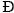The Australian Journal of Mathematical Analysis and Applications

 Home News Editors Volumes RGMIA Subscriptions Authors Contact

ISSN 1449-5910

You searched for horwitz
Total of 10 results found in site

5: Paper Source PDF document

Paper's Title:

Ellipses Inscribed in Parallelograms

Author(s):

A. Horwitz

Penn State University,
25 Yearsley Mill Rd.
Media, PA 19063
U. S. A.
alh4@psu.edu

Abstract:

We prove that there exists a unique ellipse of minimal eccentricity, EI, inscribed in a parallelogram, Đ. We also prove that the smallest nonnegative angle between equal conjugate diameters of \$EI equals the smallest nonnegative angle between the diagonals of Đ. We also prove that if EM is the unique ellipse inscribed in a rectangle, R, which is tangent at the midpoints of the sides of R, then EM is the unique ellipse of minimal eccentricity, maximal area, and maximal arc length inscribed in R. Let Đ be any convex quadrilateral. In previous papers, the author proved that there is a unique ellipse of minimal eccentricity, EI, inscribed in Đ, and a unique ellipse, EO, of minimal eccentricity circumscribed about Đ. We defined Đ to be bielliptic if EI and EO have the same eccentricity. In this paper we show that a parallelogram, Đ, is bielliptic if and only if the square of the length of one of the diagonals of Đ equals twice the square of the length of one of the sides of Đ .

3: Paper Source PDF document

Paper's Title:

Ellipses of Maximal Area and of Minimal Eccentricity Inscribed in a Convex Quadrilateral

Author(s):

Alan Horwitz

Penn State University,
25 Yearsley Mill Rd., Media, Pa 19063
alh4@psu.edu
Url: www.math.psu.edu/horwitz

Abstract:

Let Đ be a convex quadrilateral in the plane and let M1 and M2 be the midpoints of the diagonals of Đ. It is well–known that if E is an ellipse inscribed in Đ, then the center of E must lie on Z, the open line segment connecting M1 and M2 . We use a theorem of Marden relating the foci of an ellipse tangent to the lines thru the sides of a triangle and the zeros of a partial fraction expansion to prove the converse: If P lies on Z, then there is a unique ellipse with center P inscribed in Đ. This completely characterizes the locus of centers of ellipses inscribed in Đ. We also show that there is a unique ellipse of maximal area inscribed in Đ. Finally, we prove our most signifigant results: There is a unique ellipse of minimal eccentricity inscribed in Đ.

2: Paper Source PDF document

Paper's Title:

Ellipses of Minimal Area and of Minimal Eccentricity Circumscribed About a Convex Quadrilateral

Author(s):

Alan Horwitz

Penn State University,
25 Yearsley Mill Rd.,
Media, PA 19063,
U.S.A
alh4@psu.edu

Abstract:

First, we fill in key gaps in Steiner's nice characterization of the most nearly circular ellipse which passes through the vertices of a convex quadrilateral,. Steiner proved that there is only one pair of conjugate directions, M1 and M2, that belong to all ellipses of circumscription. Then he proves that if there is an ellipse, E, whose equal conjugate diameters possess the directional constants M1 and M2, then E must be an ellipse of circumscription which has minimal eccentricity. However, Steiner does not show the existence or uniqueness of such an ellipse. We prove that there is a unique ellipse of minimal eccentricity which passes through the vertices of. We also show that there exists an ellipse which passes through the vertices ofand whose equal conjugate diameters possess the directional constants M1 and M2. We also show that there exists a unique ellipse of minimal area which passes through the vertices of. Finally, we call a convex quadrilateral,, bielliptic if the unique inscribed and circumscribed ellipses of minimal eccentricity have the same eccentricity. This generalizes the notion of bicentric quadrilaterals. In particular, we show the existence of a bielliptic convex quadrilateral which is not bicentric.

Search and serve lasted 0 second(s).